# REASONING (Test 4)

Tag: reasoning
Q.1
Which is the missing tile?A.B.C.D.Explaination / Solution:

Looking across and down, lines are carried forward from the first two squares to the final square when they appear in the same position twice in the first two squares, however, they then change from complete to broken lines and vice versa.

Workspace
Report
Q.2
A question paper had ten questions. Each question could only be answered as True (T) or False (F). Each candidate answered all the questions. Yet, no two candidates wrote the answers in an identical sequence. How many different sequences of answers are possible?
A. 20
B. 40
C. 512
D. 1024
Explaination / Solution:
No Explaination.

Workspace
Report
Q.3
Six books, A, B, C, D, E and F are placed side by side. B, C and E have blue cover and the other books have red cover. Only D and F are new books and the rest are old. A, C and D are law reports and other are Gazetteers. What book is a new law report with a red colour? Which of the statements given above is/are correct?
A. A
B. B
C. C
D. D
Explaination / Solution:
No Explaination.

Workspace
Report
Q.4
A group of 630 children is seated in rows for a group photo session. Each row contains three less children than the row in front of it. Which one of the following number of rows is not possible?
A. 3
B. 4
C. 5
D. 6
Explaination / Solution:
No Explaination.

Workspace
Report
Q.5
In a class of 45 students, a boy is ranked 20th. When two boys joined, his rank was dropped by one. What is his new rank from the end?
A. 25th
B. 26th
C. 27th
D. 28th
Explaination / Solution:
No Explaination.

Workspace
Report
Q.6
Find the number of triangles in the given figure.A. 12
B. 18
C. 22
D. 26
Explaination / Solution:

The figure may be labelled as shown.The simplest triangles are AHB, GHI, BJC, GFE, GIE, IJE, CEJ and CDE i.e. 8 in number.

The triangles composed of two components each are HEG, BEC, HBE, JGE and ICE i.e. 5 in number.

The triangles composed of three components each are FHE, GCE and BED i.e. 3 in number.

There is only one triangle i.e. AGC composed of four components.

There is only one triangle i.e. AFD composed of nine components.

Thus, there are 8 + 5 + 3 + 1 + 1 = 18 triangles in the given figure.

Workspace
Report
Q.7
Find the minimum number of straight lines required to make the given figure.A. 9
B. 11
C. 15
D. 16
Explaination / Solution:

The figure may be labelled as shown.The horizontal lines are DE, FH, IL and BC i.e. 4 in number.

The slanting lines are AC, DO, FN, IM, AB, EM and HN i.e. 7 in number.

Thus, there are 4 + 7 = 11 straight lines in the figure.

Workspace
Report
Q.8

Find the minimum number of straight lines required to make the given figure.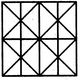A. 11
B. 14
C. 16
D. 17
Explaination / Solution:

The figure may be labelled as shown.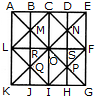The horizontal lines are AK, BJ, CI, DH and EG i.e. 5 in number.

The vertical lines are AE, LF and KG i.e. 3 in number.

The slanting lines are LC, CF, FI, LI, EK and AG i.e. 6 in number.

Thus, there are 5 + 3 + 6 = 14 straight lines in the figure.

Workspace
Report
Q.9

Count the number of squares in the given figure.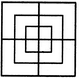A. 8
B. 12
C. 15
D. 18
Explaination / Solution:

The figure may be labelled as shown.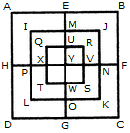The simplest squares are QUYX, URVY, YVSW and XYWT i.e. 4 in number.

The squares composed of two components each are IMYP, MJNY, YNKO and PYOL i.e. 4 in number.

The squares composed of three components each are AEYH, EBFY, YFCG and HYGD i.e. 4 in number.

There is only one square i.e. QRST composed of four components.

There is only one square i.e. IJKL composed of eight components.

There is only one square i.e. ABCD composed of twelve components.

Total number of squares in the given figure = 4 + 4 + 4+1 + 1 + 1 = 15.

Workspace
Report
Q.10

How many triangles and parallelograms are there in the following figure?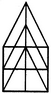A. 21, 17
B. 19, 13
C. 21, 15
D. 19, 17
Explaination / Solution:

The figure may be labelled as shown.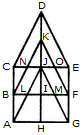Triangles:

The simplest triangles are KJN, KJO, CNB, OEF, JIL, JIM, BLA and MFG i.e. 8 in number.

The triangles composed of two components each are CDJ, EDJ, NKO, JLM, JAH and JGH i.e. 6 in number.

The triangles composed of three components each are BKI, FKI, CJA and EJG i.e. 4 in number.

The triangles composed of four components each are CDE and AJG i.e. 2 in number.

The only triangle composed of six components is BKF.

Thus, there are 8 + 6 + 4 + 2 + 1 = 21 triangles in the given figure.

Parallelograms :

The simplest parallelograms are NJLB and JOFM i.e. 2 in number.

The parallelograms composed of two components each are CDKB, DEFK, BIHA and IFGH i.e.4 in number.

The parallelograms composed of three components each are BKJA, KFGJ, CJIB and JEFI i.e.4 in number.

There is only one parallelogram i.e. BFGA composed of four components.

The parallelograms composed of five components each are CDJA, DEGJ, CJHA and JEGH i.e.4 in number.

The only parallelogram composed of six components is CEFB.

The only parallelogram composed of ten components is CEGA.

Thus, there are 2 + 4 + 4 + 1 + 4+ 1 + 1 = 17 parallelograms in the given figure.

(Here note that the squares and rectangles are also counted amongst the parallelograms).

Workspace
Report